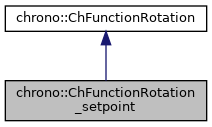chrono::ChFunctionRotation_setpoint Class Reference

## Description

A rotation q=f(s) provided from a rotation sample, continuously updated by the user, behaving as a ZOH (zero order hold) of FOH (first order).

You must keep the setpoint q updated via multiple calls to SetSetpoint(), for example calling SetSetpoint() at each timestep in the simulation loop. It is assumed that one will later evaluate Get_q(), Get_w_loc() etc. in close vicinity of the setpoint (setpoint history is not saved), preferably ecactly at the same s, but in vicinity of s there will be extrapolation (after last s) and interpolation (before last s) according to: If in ZOH mode: rotation q will persist indefinitely until next call, angular velocity and angular acceleration will be zero. If in FOH mode: rotation q will interpolate linearly from the previous value, angular velocity will be constant, angular acceleration will be zero. Default: uses FOH mode. Use SetMode() to change it.

#include <ChFunctionRotation_setpoint.h>

Inheritance diagram for chrono::ChFunctionRotation_setpoint:[legend]
Collaboration diagram for chrono::ChFunctionRotation_setpoint:[legend]

## Public Types

enum  eChSetpointMode { ZOH, FOH, OVERRIDE }
Type of setpoint interpolation/extrapolation - zero order hold, first order hold, etc. More...

## Public Member Functions

ChFunctionRotation_setpoint (const ChFunctionRotation_setpoint &other)

virtual ChFunctionRotation_setpointClone () const override
"Virtual" copy constructor.

void SetMode (eChSetpointMode mmode)
Sets the extrapolation/interpolation mode.

eChSetpointMode GetMode ()
Gets the extrapolation/interpolation mode.

void Reset (double ms=0)
Use this to go back to s=0 (the SetSetpoint() function works only if called at increasing s values)

virtual void SetSetpoint (ChQuaternion<> q_setpoint, double s)
Set the rotation setpoint, and compute its derivatives (angular speed, angular acceleration) automatically by backward differentiation (only if s is called at increasing small steps, most often s is time). More...

virtual void SetSetpointAndDerivatives (ChQuaternion<> q_setpoint, ChVector<> w_loc_setpoint, ChVector<> a_loc_setpoint)
Set the setpoint, and also its derivatives (angular velocity, angular acceleration). More...

virtual ChQuaternion Get_q (double s) const override
Return the q value of the function, at s, as q=f(s).

virtual ChVector Get_w_loc (double s) const override
Return the derivative of the rotation function, at s, expressed as angular velocity w in local frame.

virtual ChVector Get_a_loc (double s) const override
Return the derivative of the rotation function, at s, expressed as angular acceleration in local frame.

virtual void ArchiveOut (ChArchiveOut &marchive) override
Method to allow serialization of transient data to archives.

virtual void ArchiveIn (ChArchiveIn &marchive) override
Method to allow de-serialization of transient data from archives.Public Member Functions inherited from chrono::ChFunctionRotation
ChFunctionRotation (const ChFunctionRotation &other)

virtual void Estimate_s_domain (double &smin, double &smax) const
Return an estimate of the domain of the function argument. More...

virtual void Update (const double t)
Update could be implemented by children classes, ex. to launch callbacks.

## ◆ eChSetpointMode

Type of setpoint interpolation/extrapolation - zero order hold, first order hold, etc.

Enumerator
ZOH

Zero Order Hold: q constant, w = a = 0 in neighbour of setpoint s.

FOH

First Order Hold: q linear, w constant, a = 0 in neighbour of setpoint s.

OVERRIDE

p, w, a are set via SetSetpointAndDerivatives() and will be considered constant in Get_q(s) regardless of s until next SetSetpointAndDerivatives()

## ◆ SetSetpoint()

 void chrono::ChFunctionRotation_setpoint::SetSetpoint ( ChQuaternion<> q_setpoint, double s )
virtual

Set the rotation setpoint, and compute its derivatives (angular speed, angular acceleration) automatically by backward differentiation (only if s is called at increasing small steps, most often s is time).

Note: each time must be called with increasing s so that internally it add sthe 'new' setpoint and scrolls the previous samples for computing extrapolation/interpolation, but if called multiple times with exactly the same s value, the buffer of past samples is not scrolled: it just recompute setpoint and derivatives according to the 'updated' setpoint. If in ZOH mode: rotation q will persist indefinitely until next call, angular velocity and angular acceleration will be zero. If in FOH mode: rotation q will interpolate linearly from the previous value, angular velocity will be constant, angular acceleration will be zero.

## ◆ SetSetpointAndDerivatives()

 virtual void chrono::ChFunctionRotation_setpoint::SetSetpointAndDerivatives ( ChQuaternion<> q_setpoint, ChVector<> w_loc_setpoint, ChVector<> a_loc_setpoint )
inlinevirtual

Set the setpoint, and also its derivatives (angular velocity, angular acceleration).

Moreover, changes the mode to eChSetpointMode::OVERRIDE, so all values will persist indefinitely until next call, that is multiple calls to Get_q(s) Get_w_loc() etc. will give same results (non interpolated) regardless of s.

The documentation for this class was generated from the following files:
• /builds/uwsbel/chrono/src/chrono/motion_functions/ChFunctionRotation_setpoint.h
• /builds/uwsbel/chrono/src/chrono/motion_functions/ChFunctionRotation_setpoint.cpp